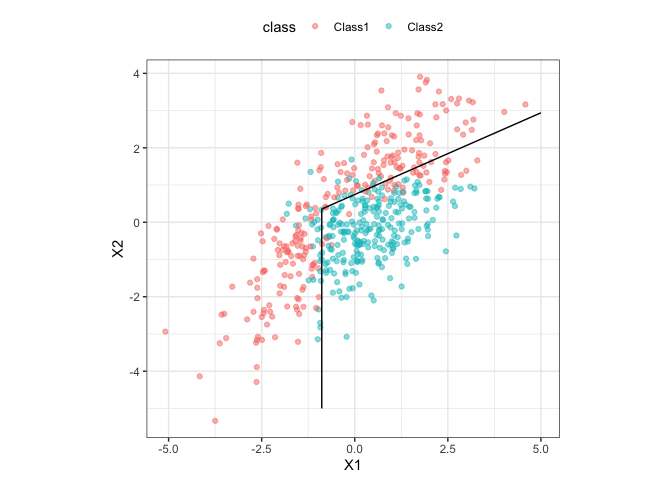discrim contains simple bindings to enable the parsnip package to fit various discriminant analysis models, such as

• Linear discriminant analysis (LDA, simple and regularized)
• Quadratic discriminant analysis (QDA, simple and regularized)
• Regularized discriminant analysis (RDA, via Friedman (1989))
• Flexible discriminant analysis (FDA) using MARS features
• Naive Bayes models

## Installation

You can install the released version of discrim from CRAN with:

install.packages("discrim")

And the development version from GitHub with:

# install.packages("pak")
pak::pak("tidymodels/discrim")

## Available Engines

The discrim package provides engines for the models in the following table.

model engine mode
discrim_flexible earth classification
discrim_linear MASS classification
discrim_linear mda classification
discrim_linear sda classification
discrim_linear sparsediscrim classification
discrim_regularized klaR classification
naive_Bayes klaR classification
naive_Bayes naivebayes classification

## Example

Here is a simple model using a simulated two-class data set contained in the package:

library(discrim)

parabolic_grid <-
expand.grid(X1 = seq(-5, 5, length = 100),
X2 = seq(-5, 5, length = 100))

fda_mod <-
discrim_flexible(num_terms = 3) %>%
# increase num_terms to find smoother boundaries
set_engine("earth") %>%
fit(class ~ ., data = parabolic)

parabolic_grid$fda <- predict(fda_mod, parabolic_grid, type = "prob")$.pred_Class1

library(ggplot2)
ggplot(parabolic, aes(x = X1, y = X2)) +
geom_point(aes(col = class), alpha = .5) +
geom_contour(data = parabolic_grid, aes(z = fda), col = "black", breaks = .5) +
theme_bw() +
theme(legend.position = "top") +
coord_equal()## Contributing

This project is released with a Contributor Code of Conduct. By contributing to this project, you agree to abide by its terms.